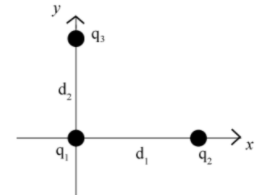# Problem: Three charges are located as shown in the figure, with values q1 = 4.9x10-16 C, q2 = -1.5x10-16 C, q3 = 6.75x10-16 C. The charges are separated by d1 = 3.9x10-6 m and d2 = 3.9x10-6 m.a. What is the force of q2 on q1 in the x direction, Fx? Give your answer in newtons, and recall k = 8.988 × 109 N m2/C2.b. What is the force of q3 on q1 in the y direction, Fy? Give your answer in newtons.

###### FREE Expert Solution

We'll determine the forces from Coulomb's law given by:

$\overline{){\mathbf{F}}{\mathbf{=}}\frac{\mathbf{k}{\mathbf{q}}_{\mathbf{1}}{\mathbf{q}}_{\mathbf{2}}}{{\mathbf{r}}^{\mathbf{2}}}}$

a.

The force of q2 on q1 in the x-direction is:

${{\mathbf{F}}}_{{\mathbf{x}}}{\mathbf{=}}\frac{{\mathbf{kq}}_{\mathbf{1}}{\mathbf{q}}_{\mathbf{2}}}{{{\mathbf{d}}_{\mathbf{1}}}^{\mathbf{2}}}$

k = 8.988 × 109 N m2/C2

97% (1 ratings)###### Problem Details

Three charges are located as shown in the figure, with values q1 = 4.9x10-16 C, q2 = -1.5x10-16 C, q3 = 6.75x10-16 C. The charges are separated by d1 = 3.9x10-6 m and d2 = 3.9x10-6 m.a. What is the force of q2 on q1 in the x direction, Fx? Give your answer in newtons, and recall k = 8.988 × 109 N m2/C2.

b. What is the force of q3 on q1 in the y direction, Fy? Give your answer in newtons.

Frequently Asked Questions

What scientific concept do you need to know in order to solve this problem?

Our tutors have indicated that to solve this problem you will need to apply the Coulomb's Law (Electric Force) concept. You can view video lessons to learn Coulomb's Law (Electric Force). Or if you need more Coulomb's Law (Electric Force) practice, you can also practice Coulomb's Law (Electric Force) practice problems.

What professor is this problem relevant for?

Based on our data, we think this problem is relevant for Professor Vallone's class at BROOKLYN CUNY.